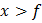# An infinitely long rod lies along the axis of concave mirror of focal lengthThe near end of the rod is at a distancefrom the mirror. Then the length of the image of the rod is a)b)c)d)## Question ID - 152503 :- An infinitely long rod lies along the axis of concave mirror of focal lengthThe near end of the rod is at a distancefrom the mirror. Then the length of the image of the rod is a)b)c)d)3537

 (d)andUsing above relation, the length of image